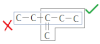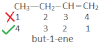# Naming Of Organic Compounds Containing Multiple Bonds

1. Select longest continuous chain of c-atom containing multiple bonds.1. If only one multiple bonds is present (either double or triple) then C-atom form where multiple bond begins, gets the lowest position number.1. If one type of multiple bond present more than one time, numbering should be done in a way such that the sum of locants would be lowest.
CH2=CH-CH=CH-CH3
Penta-2, 4-diene (wrong)
Penta-1, 3-diene (correct)

2. If both double and triple bond are present at equal position form the two ends then the double bond containing C-atom should get the lowest position number.
CH≡C-CH2-CH=CH2 (pent-1-en-4-yne)